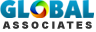# Brief overview of Pin-jointed Truss and different Analysis methodsTruss belongs to a structure that contains straight members to build up one or more triangular units. Generally Pin-joints are used to tie the members of truss at the end. The joints concerning a truss are defined as nodes. External forces and reactions can only function at the nodes and produce forces in the members to be axial forces (tensile or compressive).

If all the members and nodes are positioned inside a two dimensional plane, it is called plane truss. If a truss consists of members and nodes and expands into three dimensions, it is called space truss.

Trusses are inherent parts of various structures like bridges, roof supports, transmission towers, space stations etc.

Truss are defined as:-

a) Statically establish; all the unidentified forces (support reaction and member forces) can be specified with the use of equations of static equilibrium. (provided m+r = 2j).

b) Indeterminate; equations of static equilibrium can't solely find out unknown forces, provided m+r> 2j.

c) Unstable; not appropriate to bear load; provided m+r < 2j; Where 'm' denotes member's no in a truss; 'r' denotes number of reaction components; And 'j' denotes number of joints in a truss;

Analysis methods:

Two methods are available for determining the forces in the members concerning a truss;

(i) Methods of Joints : This method focuses on the equilibrium of the all joints concerning the truss. There exists two equations of static equilibrium ?Fx & ?Fy.So one has to start with the joint containing lower than 2 unknown forces. Go through problem 3-1 (civilengineer.webinfolist.com)

(ii) This method is useful while finding out the forces in a few members. Under this method, a fictitious is passed over the members in which forced should be set and to obtain unidentified forces, select the equilibrium of the left hand side or the right hand side of the truss.Go through problem 3-2 (civilengineer.webinfolist.com)

# Takeoff Tools